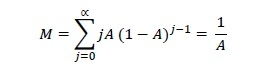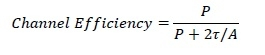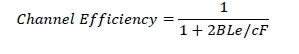# Ethernet Performance

Ethernet is a set of technologies and protocols that are used primarily in LANs. The performance of Ethernet is analysed by computing the efficiency of the channel under different load conditions.

Let us assume an Ethernet network has k stations and each station transmits with a probability p during a contention slot. Let A be the probability that some station acquires the channel. A is calculated as −

A = kp (1−p)kp

The value of A is maximized at p = 1/k. If there can be innumerable stations connected to the Ethernet network, i.e. k → ∞, the maximum value of A will be 1/e.

Let Q be the probability that the contention period has exactly j slots. Q is calculated as −

Q = A (1−A)j−1

Let M be the mean number of slots per contention. So, the value of M will be −Given that τ is the propagation time, each slot has duration 2τ. Hence the mean contention interval, 𝑤 will be 2τ/A.

Let P be the time is seconds for a frame to propagate.

The channel efficiency, when a number of stations want to send frame, can be calculated as −Let F be the length of frame, B be the cable length, L be the cable length, c be the speed of signal propagation and e be the contention slots per frame. The channel efficiency in terms of these parameters is −